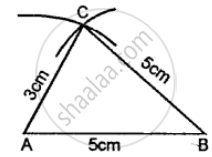# Construct Traingle Abc, When : Ab = Bc = 5 Cm and Ac = 3 Cm. Mea¬Sure Angles a and C. is ∠A = ∠C? - Mathematics

Diagram
Sum

Construct traingle ABC, when : AB = BC = 5 cm and AC = 3 cm. Mea¬sure angles A and C. Is ∠A = ∠C?

#### Solution

Steps of Construction:

(1) Draw a line AB = 5 cm.(2) Using compasses and taking B as cen¬tre, draw an arc of 5 cm radius.

(3) With A as a centre, draw an arc of 3 cm radius, which cuts the previous arc at C.

(4) Join AC and BC.

Concept: Classification of Triangles (On the Basis of Sides, and of Angles)
Is there an error in this question or solution?

#### APPEARS IN

Selina Class 6 Mathematics
Chapter 26 Triangles (Including Types, Properties and Constructions)
Exercise 26 (B) | Q 3